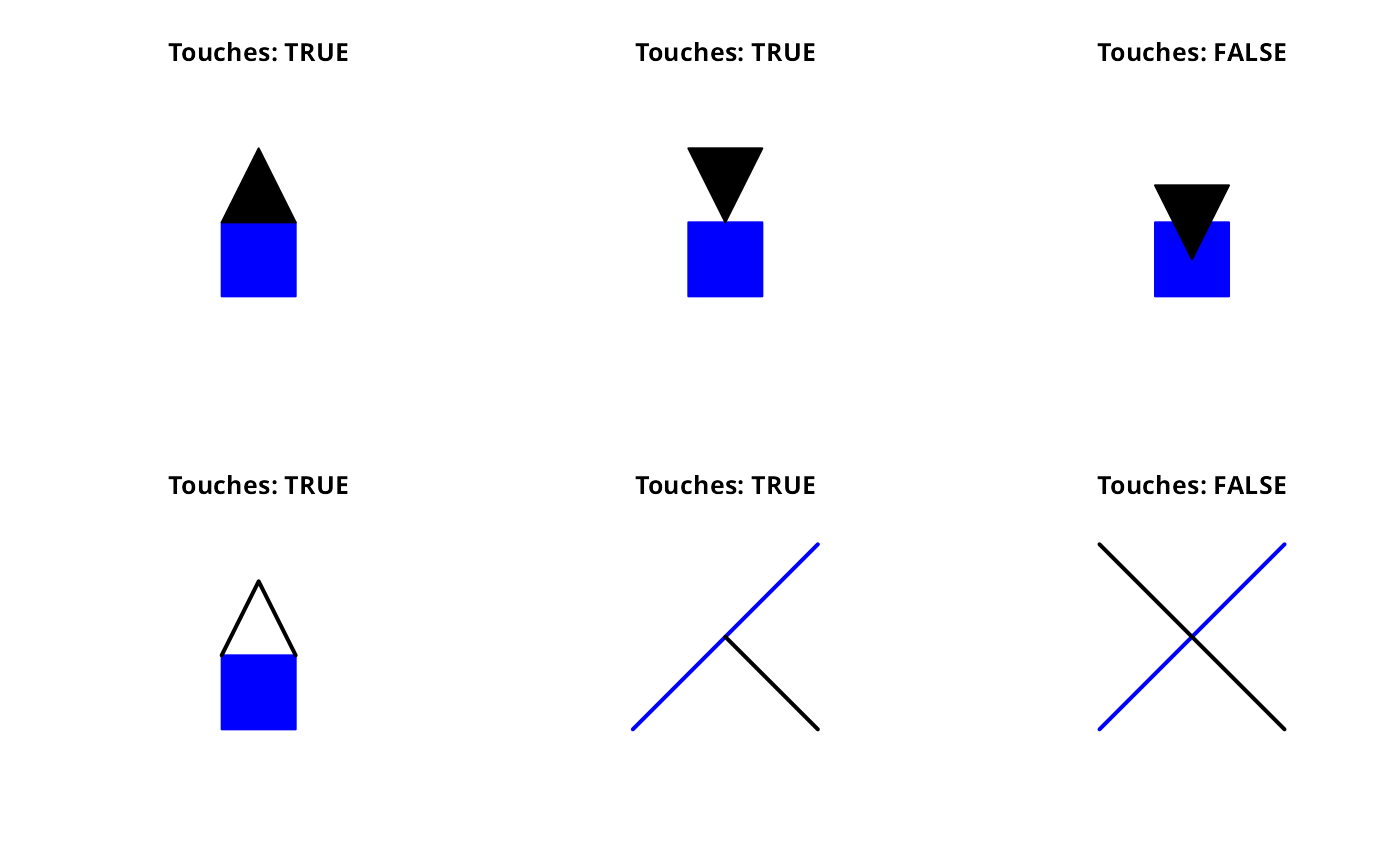Functions for testing if the geometries have at least one boundary point in common, but no interior points

gTouches(spgeom1, spgeom2 = NULL, byid = FALSE, returnDense=TRUE,
checkValidity=FALSE)

## Arguments

spgeom1, spgeom2

sp objects as defined in package sp. If spgeom2 is NULL then spgeom1 is compared to itself.

byid

Logical vector determining if the function should be applied across ids (TRUE) or the entire object (FALSE) for spgeom1 and spgeom2

returnDense

default TRUE, if false returns a list of the length of spgeom1 of integer vectors listing the 1:length(spgeom2) indices which would be TRUE in the dense logical matrix representation; useful when the sizes of the byid=TRUE returned matrix is very large and it is sparse; essential when the returned matrix would be too large

checkValidity

default FALSE; error meesages from GEOS do not say clearly which object fails if a topology exception is encountered. If this argument is TRUE, gIsValid is run on each in turn in an environment in which object names are available. If objects are invalid, this is reported and those affected are named

## Value

Returns TRUE if the intersection of the boundaries of the two geometries is not empty.

## Note

Error messages from GEOS, in particular topology exceptions, report 0-based object order, so geom 0 is spgeom1, and geom 1 is spgeom2.

## Author

Roger Bivand & Colin Rundel

gContains gContainsProperly gCovers gCoveredBy gCrosses gDisjoint gEquals gEqualsExact gIntersects gOverlaps gRelate gWithin

## Examples


p1 = readWKT("POLYGON((0 0,1 0,1 1,0 1,0 0))")
p2 = readWKT("POLYGON((0 1,0.5 2,1 1,0 1))")
p3 = readWKT("POLYGON((0.5 1,0 2,1 2,0.5 1))")
p4 = readWKT("POLYGON((0.5 0.5,0 1.5,1 1.5,0.5 0.5))")

l0 = readWKT("LINESTRING(0 1,0.5 2,1 1)")

par(mfrow=c(2,3))
title(paste("Touches:",gTouches(p1,p2)))

title(paste("Touches:",gTouches(p1,p3)))